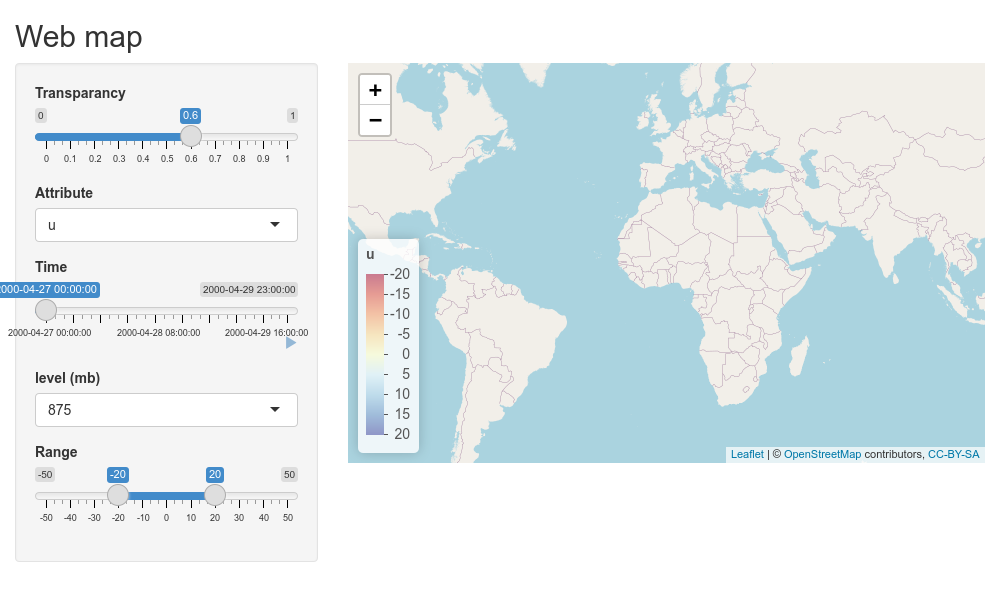# Using tiles in shiny

In this manual we will discuss an example of using starsTileServer for serving map tiles in a shiny app. It shows the ability to visualize different layers and dynamically change layers. To do this we will use a sample dataset from the ERA5 weather model, that includes different variables and pressure levels.

library(starsTileServer)

The sample dataset can be retrieved with the following code using the ecmwfr R package:

require(ecmwfr)
request <-
list(
"dataset_short_name" = "reanalysis-era5-pressure-levels",
"product_type" = "reanalysis",
"variable" = c("temperature", "geopotential", "u_component_of_wind", "v_component_of_wind"),
"pressure_level" = c("875", "900", "925"),
"year" = "2000",
"month" = "04",
"day" = as.character(27:29),
"time" = sprintf("%02i:00", 0:23),
"area" = "64/-130/-64/144",
"format" = "netcdf",
"target" = "ecmwfData.nc"
)
# make sure you use your own uid and key ( https://cds.climate.copernicus.eu/#!/home )
wf_set_key("uid_to_replace", "key_to_replace", service = "cds")
ncfile <- wf_request(
user = "uid_to_replace",
request = request,
transfer = TRUE,
path = "~",
verbose = FALSE
)

## Starting the tileserver

To set up the tile server we need the grid file loaded. Additionally a color function can be added. This function needs to have the same format as the color as the color mapping functions in leaflet.

weatherData <- stars::read_stars(tmpGridFile)
#> t, z, u, v,
sf::st_crs(weatherData) <- "+proj=longlat"

colFun <- function(x,
alpha = 1,
max = 20,
min = -20) {
paste0(
suppressWarnings(leaflet::colorNumeric(
"RdYlBu",
domain = c(as.numeric(min), as.numeric(max))
)(x)),
as.character(as.raw(as.numeric(alpha) * 255))
)
}
attr(colFun, "colorType") <- "numeric"

The tileserver needs to run in a separate process. On a personal computer this can easily be achieved by running two R processes at the same time. An alternative approach is to use callr to start a separate process.

# note the process is ran in the background, do not forget to close it as it might use quite a bit of memory.
# I have made the experience that callr seems to work poorly if the process is rather verbose
require(callr)
rp <- r_bg(args = list(grid = weatherData, colFun = colFun), function(grid, colFun) {
starsTileServer::starsTileServer$new(grid, colFun)$run(port = 3746, docs = TRUE)
})

The process will print an url where documentation an testing for the server is available:

message(rp$read_error()) #> Running plumber API at http://127.0.0.1:3746 #> Running swagger Docs at http://127.0.0.1:3746/__docs__/ # Shiny app Now a small example of some interaction based on a shiny example. First we create UI, this consists of a few selection options to change the map features. require(shiny) #> Loading required package: shiny require(leaflet) require(stars) #> Loading required package: stars #> Loading required package: abind #> Loading required package: sf #> Linking to GEOS 3.8.0, GDAL 3.0.4, PROJ 6.3.1; sf_use_s2() is TRUE weather <- stars::read_stars(tmpGridFile, proxy = T) #> t, z, u, v, ui <- fluidPage( # Application title titlePanel("Web map"), sidebarLayout( # Sidebar with a slider input sidebarPanel( sliderInput("alpha", "Transparancy", 0, 1, .6, .01), selectInput("attr", "Attribute", choices = c("u", "v")), sliderInput( "time", "Time", value = min(st_get_dimension_values(weather, 4)), min = min(st_get_dimension_values(weather, 4)), max = max(st_get_dimension_values(weather, 4)), step = 3600, timezone = "+0000", animate = animationOptions(5000) ), selectInput("level", "level (mb)", choices = as.character(st_get_dimension_values(weather, 3))), sliderInput("colRange", "Range", -50, 50, c(-20, 20)) ), # Show a plot of the generated distribution mainPanel(leafletOutput("map")) ) ) We use the following shiny server function: server <- function(input, output, session) { # This reactive creates the URL to the tileserver, it include the different input variables in requests to the server # The debounce ensures the URL does not get updated to frequent url <- reactive({ sprintf( "http://127.0.0.1:3746/map/%s/{z}/{x}/{y}?level=%s&alpha=%f&time=%s&min=%f&max=%f", input$attr,
input$level, input$alpha,
strftime(input$time, tz = "UTC", format = "%Y-%m-%d %H:%M:%S"), min(input$colRange),
max(input$colRange) ) }) %>% debounce(100) # This reactive downloads the color function from the server and prepares it for adding as a legend to the leaflet map colorFunction <- reactive({ f <- readRDS(base::url(sprintf("http://127.0.0.1:3746/map/%s/colorfunctionnoalpha", input$attr)))
at <- attributes(f)
if (is.finite(max(colrange()))) {
formals(f)$max <- max(colrange()) } if (is.finite(min(colrange()))) { formals(f)$min <- min(colrange())
}
attributes(f) <- at
f
})
colrange <- reactive(range(input$colRange)) output$map <- renderLeaflet({
leaflet() %>%
fitBounds(-50, -30, 50, 50)
})
# This observer changes the tile layer as soon as the url is updated
observe({
leafletProxy("map") %>%
clearGroup("wind") %>%
title = input$attr, position = "bottomleft" ) }) } The app can be create using the regular shiny functionality app <- shinyApp(ui, server) The result of this shiny app looks as follows:To wrap up we close the tile server rp$finalize()